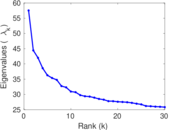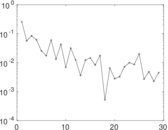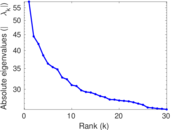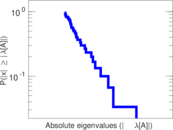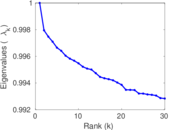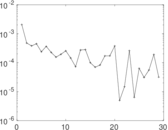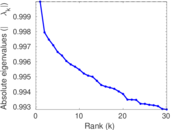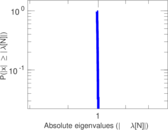# IMDB

These are persons associated with individual movies or television programs. The network is bipartite. Left nodes are persons (actors, directors, etc.), and right nodes are works (films, television programs, etc.). An edge denotes that the person was involved in the work.

 Code `IM` Internal name `komarix-imdb` Name IMDB Data source http://komarix.org/ac/ds/ AvailabilityDataset is available for download Consistency checkDataset passed all tests Category Affiliation network Node meaning Person, work Edge meaning Association Network formatBipartite, undirected Edge typeUnweighted, no multiple edges

## Statistics

 Size n = 871,982 Left size n1 = 685,568 Right size n2 = 186,414 Volume m = 2,715,604 Wedge count s = 86,342,427 Claw count z = 3,510,393,982 Square count q = 11,803,894 4-Tour count T4 = 445,236,096 Maximum degree dmax = 1,293 Maximum left degree d1max = 654 Maximum right degree d2max = 1,293 Average degree d = 6.228 58 Average left degree d1 = 3.961 10 Average right degree d2 = 14.567 6 Size of LCC N = 859,975 Diameter δ = 34 50-Percentile effective diameter δ0.5 = 6.950 99 90-Percentile effective diameter δ0.9 = 8.862 68 Median distance δM = 7 Mean distance δm = 7.321 59 Gini coefficient G = 0.715 542 Balanced inequality ratio P = 0.204 069 Left balanced inequality ratio P1 = 0.234 662 Right balanced inequality ratio P2 = 0.320 584 Relative edge distribution entropy Her = 0.931 840 Power law exponent γ = 2.043 11 Tail power law exponent γt = 3.761 00 Degree assortativity ρ = −0.047 564 0 Degree assortativity p-value pρ = 0.000 00 Spectral norm α = 57.531 6 Spectral separation |λ1[A] / λ2[A]| = 1.294 69 Controllability C = 527,541 Relative controllability Cr = 0.604 991

## Plots

### Fruchterman–Reingold graph drawing### Degree distribution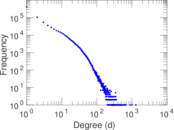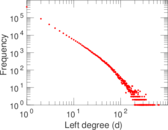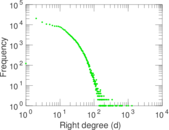### Cumulative degree distribution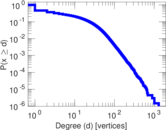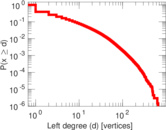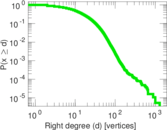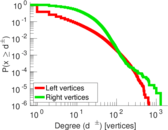### Lorenz curve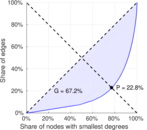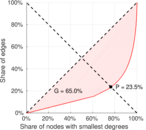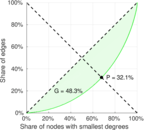### Spectral distribution of the adjacency matrix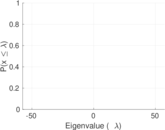### Spectral distribution of the normalized adjacency matrix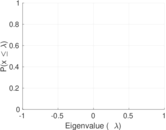### Spectral distribution of the Laplacian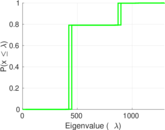### Spectral graph drawing based on the adjacency matrix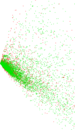### Spectral graph drawing based on the normalized adjacency matrix### Degree assortativity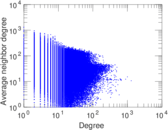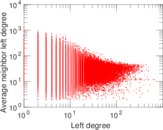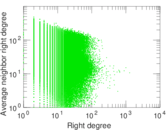### Zipf plot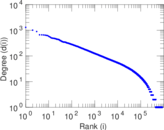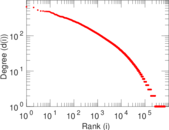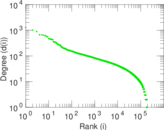### Hop distribution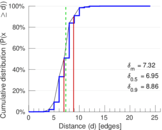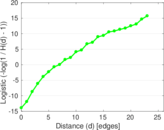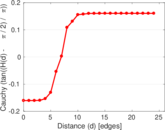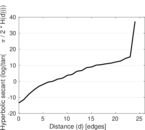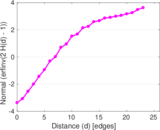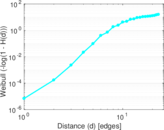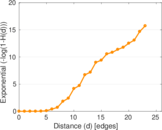### Delaunay graph drawing### Matrix decompositions plots